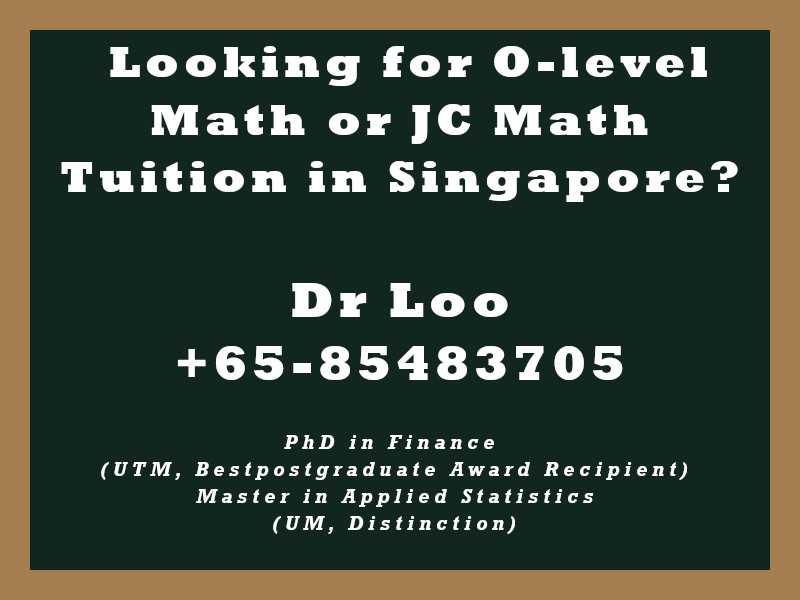+65-85483705
Select Page

# Trigonometric Identities

### Quotient Identities

• tanx = sinx/cosx
• cotx = cosx/sinx

### Double Angle Formulas

• sin2x = 2sinxcosx
• cos2x = cos2x – sin2x / 2cos2x – 1 / 1-2sin2x

### Reciprocal Identities

• sin x = 1/cosec x
• cos x = 1/sec x
• tan x = 1/cot x

### Pythagorean Identities

• sin2x + cos2x = 1
• 1+tan2x = sec2x
• 1+cot2x = cosec2x

Which can be derived from Pythagoras’ Theorem.

### Sum and Difference formulas

• sin(x+y) = sin x(cos y) + cos x(sin y)
• cos(x+y) = cos x(cos y) – sin x(sin y)
• sin(x-y) = sin x(cos y) – cos x(sin y)
• cos(x-y) = cos x(cos y) + sin x(sin y)

## About the Math Tutor - Dr Loo

I am a PhD holder with 9 years of teaching experience at secondary school and university. My expertizes are mathematics, statistics, econometrics, finance and machine learning.

Currently I do provide consultation and private tuition in Singapore. For those who are looking for math tutor in Singapore or statistics tutor in Singapore, please feel free to contact me at +65-85483705 (SMS/Whatsapp/Telegram).

Some of the math tuition in Singapore I provide includes O-level math tuition and also JC Math Tuition (H1 Math Tuition & H2 Math Tuition). On the other hand, my statistics tuition in Singapore mostly focus on pre-university level until postgraduate level. For those who prefer online tutoring service, the online O-level math tuition and online JC math tuition are also available.## For those who are looking for math tution in Singapore

Need help with this topic? I do provide mathematics home tuition in Singapore for O-level math and also JC H2 math. In addition, online math tutoring is available as well. Feel free to contact me if you would like to know further.